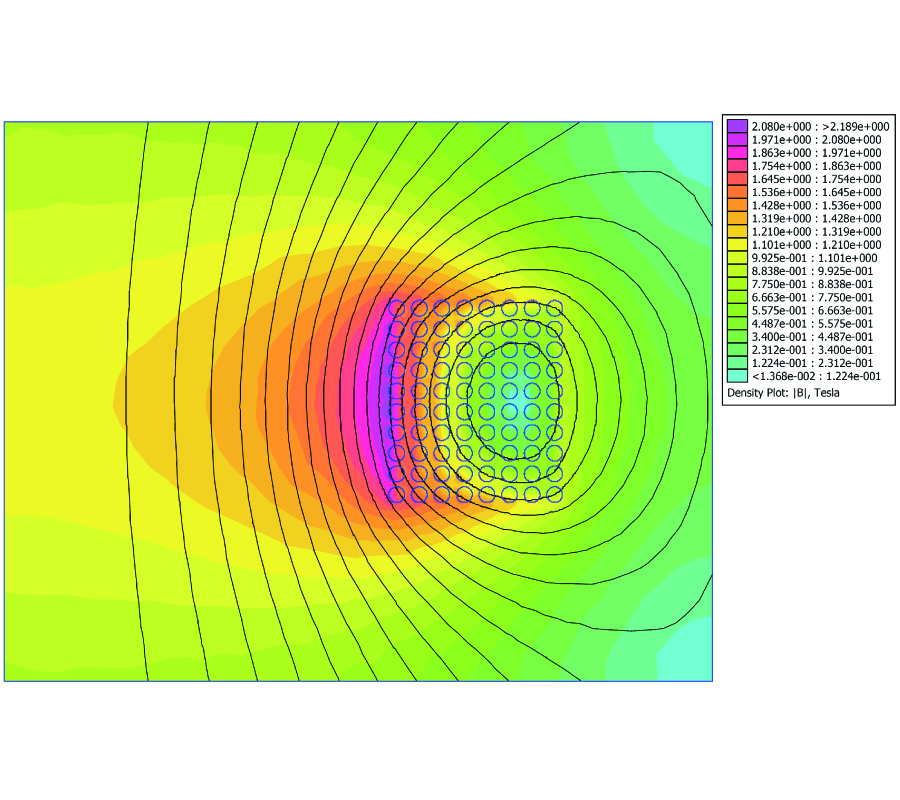# Vol. 20

Front:[PDF file] Back:[PDF file]
Latest Volume
All Volumes
All Issues
2010-04-22

#### Inductance Comparison of the Solenoidal Coil of Modular Toroidal Coils Using the Analytical and Finite Element Method

By Mohammad Reza Pahlavani, Abbas Shiri, H. A. Mohammadpour, and Abbas Shoulaie
Progress In Electromagnetics Research B, Vol. 20, 337-352, 2010
doi:10.2528/PIERB10030907

## Abstract

The modular toroidal coil (MTC) is composed of several solenoidal coils (SCs) connected in series and distributed in toroidal and symmetrical forms. In this paper, we present an accurate approach for calculation of the mutual and self-inductance between all the SCs of MTC with any arbitrary section. We use Biot-Savart's and Neumann's equations to calculate the self- and mutual inductance between two filamentary circular rings with inclined axes that lie in the same plane, respectively. Their centers are either displaced along the axis of one coil or displaced along one axis of the first coil and then displaced sideways. We use the extended three-point Gaussian algorithm to solve the numerical analysis of the integrations resulting from these equations. Additionally, we apply the filament method to calculate the inductance of the MTC coil. Moreover, the finite element method (FEM) is employed to obtain SC inductance. The results obtained using the FEM confirms the analytical and empirical results. Furthermore, the comparison of the behavior of SC inductance, when the dimensional parameters of the SC are changed, with the FEM results shows an error of less than 0.2%. In this approach, we clarify how the presented equations have to be used for different coil combinations in the filament treatment. Thus, the presented approach can be easily used to calculate the mutual and self-inductance of a MTC between any two MTC rings in three dimensions.

## Citation

Mohammad Reza Pahlavani, Abbas Shiri, H. A. Mohammadpour, and Abbas Shoulaie, "Inductance Comparison of the Solenoidal Coil of Modular Toroidal Coils Using the Analytical and Finite Element Method," Progress In Electromagnetics Research B, Vol. 20, 337-352, 2010.
doi:10.2528/PIERB10030907
http://jpier.org/PIERB/pier.php?paper=10030907

## References

1. Alizadeh Pahlavani, M. R. and A. Shoulaie, "A novel approach for calculations of helical toroidal coils inductance usable in reactor plasmas ," IEEE Trans. on Plasma Science, Vol. 37, No. 8, 1593-1603, Aug. 2009.
doi:10.1109/TPS.2009.2023548

2. Alizadeh Pahlavani, M. R. and A. Shoulaie, "Elimination of compressive stress in helical toroidal coils advanced structure for reactor plasmas," IEEE Trans. on Plasma Science, Vol. 37, No. 11, 2166-2177, Nov. 2009.
doi:10.1109/TPS.2009.2028637

3. Alizadeh Pahlavani, M. R. and A. Shoulaie, "Behavioral study of stress in toroidal, solenoidal, and helical toroidal coils with similar ring structures for reactor plasma," International Review of Electrical Engineering, Vol. 4, No. 1, 146-158, Jan.-Feb. 2009.

4. Romeo, A. and R. P. Garabedian, "Choice of coils for a fusion reactor," Proceedings of the National Academy of Sciences, Vol. 104, No. 30, 12250-12252, Jul. 24, 2007.

5. Babic, S. I. and C. Akyel, "New mutual inductance calculation of the magnetically coupled coils: Thin disk coil-thin wall solenoid," Journal of Electromagnetic Waves and Applications, Vol. 20, No. 10, 1281-1290, 2006.
doi:10.1163/156939306779276794

6. Akyel, C., S. I. Babic, and M.-M. Mahmoudi, "Mutual inductance calculation for non-coaxial circular air coils with parallel axes," Progress In Electromagnetics Research, Vol. 91, 287-301, 2009.
doi:10.2528/PIER09021907

7. Akyel, C., S. I. Babic, S. Kincic, and J. P. Lagace, "Magnetic force calculation between thin circular coils and thin filamentary circular coil in air," Journal of Electromagnetic Waves and Applications, Vol. 21, No. 9, 1273-1283, 2007.

8. Shiri, A. and A. Shoulaie, "A new methodology for magnetic orce calculations between planar spiral coils," Progress In Electromagnetics Research, Vol. 95, 39-57, 2009.
doi:10.2528/PIER09031608

9. Koledintseva, M. Y., J. L. Drewniak, T. P. Van Doren, D. J. Pommerenke, M. Cocchini, and D. M. Hockanson, "Method of edge currents for calculating mutual external inductance in a microstrip structure," Progress In Electromagnetics Research, Vol. 80, 197-224, 2008.
doi:10.2528/PIER07101504

10. Koledintseva, M. Y., J. L. Drewniak, T. P. Van Doren, D. J. Pommerenke, M. Cocchini, and D. M. Hockanson, "Mutual external inductance in stripline structures," Progress In Electromagnetics Research, Vol. 80, 349-368, 2008.
doi:10.2528/PIER07111503

11. Ravaud, R., G. Lemarquand, and V. Lemarquand, S. I. Babic, C. Akyel, "Mutual inductance and force exerted between thick coils ," Progress In Electromagnetics Research, Vol. 102, 367-380, 2010.

12. Zhao, P. and H.-G. Wang, "Resistances and inductances extraction using surface integral equation with the acceleration of multilevel Green function interpolation method ," Progress In Electromagnetics Research, Vol. 83, 43-54, 2008.
doi:10.2528/PIER08032001

13. Wang, H.-G. and P. Zhao, "Combining multilevel Green's function interpolation method with volume loop bases for inductance extraction problems," Progress In Electromagnetics Research, Vol. 80, 225-239, 2008.
doi:10.2528/PIER07102101

14. Tang, W., X. He, T. Pan, and Y. L. Chow, "Synthetic asymptote formulas of equivalent circuit components of square spiral inductors," Journal of Electromagnetic Waves and Applications, Vol. 20, No. 2, 215-226, 2006.
doi:10.1163/156939306775777206

15. G., M. W. McCall, D. Censor, "The theory of low-frequency wave physics revisited," Journal of Electromagnetic Waves and Applications, Vol. 21, No. 2, 229-249, 2007.
doi:10.1163/156939307779378763

16. Pennington, R. H., Introductory Computer Methods and Numerical Analysis, 4th edition, Macmillan, New York, 1970.

17. Bueno, M. and A. K. T. Assis, Equivalence between the Formulas for Inductance Calculation, 357-362, NRC, Canada, 1997.

18. Wheeler, H. A., "Formulas for the skin effect," Proceedings of the I.R.E., 412-424, Sep. 1942.
doi:10.1109/JRPROC.1942.232015

19. Grover, F. W., Inductance Calculation Working Formulas and Tables, 77-87, Dover Publications, Inc, New York, 1946.

20. Maxwell, J. C., "A treatise on Electricity and Magnetism,", Vol. 1, 297, (reprint from the original from 1873, 693), Dover Publications Inc, New York, 1954.

21. Anderson, D. A., J. C. Tannehill, and R. H. Pletcher, Computational Fluid Mechanics and Heat Transfer, Hemisphere, Washington, DC, 1984.